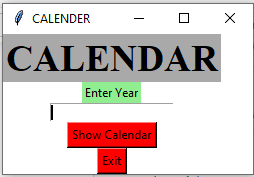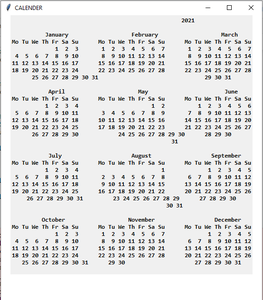Related Articles
Python | GUI Calendar using Tkinter
• Difficulty Level : Easy
• Last Updated : 19 Jan, 2021

Prerequisites :Introduction to tkinter

Python offers multiple options for developing a GUI (Graphical User Interface). Out of all the GUI methods, Tkinter is the most commonly used method. Python with Tkinter outputs the fastest and easiest way to create GUI applications. In this article, we will learn how to create a GUI Calendar application using Tkinter, with a step-by-step guide.

To create a tkinter :

• Importing the module – tkinter
• Create the main window (container)
• Add any number of widgets to the main window.
• Apply the event Trigger on the widgets.

The GUI would look like below:Let’s create a GUI based Calendar application that can show the calendar with respect to the given year, given by the user.

Below is the implementation :

## Python3

 `# import all methods and classes from the tkinter   ` `from` `tkinter ``import` `*`   `# import calendar module` `import` `calendar`   `# Function for showing the calendar of the given year` `def` `showCal() :`   `    ``# Create a GUI window` `    ``new_gui ``=` `Tk()` `    `  `    ``# Set the background colour of GUI window` `    ``new_gui.config(background ``=` `"white"``)`   `    ``# set the name of tkinter GUI window ` `    ``new_gui.title(``"CALENDER"``)`   `    ``# Set the configuration of GUI window` `    ``new_gui.geometry(``"550x600"``)`   `    ``# get method returns current text as string` `    ``fetch_year ``=` `int``(year_field.get())`   `    ``# calendar method of calendar module return` `    ``# the calendar of the given year .` `    ``cal_content ``=` `calendar.calendar(fetch_year)`   `    ``# Create a label for showing the content of the calender` `    ``cal_year ``=` `Label(new_gui, text ``=` `cal_content, font ``=` `"Consolas 10 bold"``)`   `    ``# grid method is used for placing ` `    ``# the widgets at respective positions ` `    ``# in table like structure.` `    ``cal_year.grid(row ``=` `5``, column ``=` `1``, padx ``=` `20``)` `    `  `    ``# start the GUI ` `    ``new_gui.mainloop()`   `    `  `# Driver Code` `if` `__name__ ``=``=` `"__main__"` `:`   `    ``# Create a GUI window` `    ``gui ``=` `Tk()` `    `  `    ``# Set the background colour of GUI window` `    ``gui.config(background ``=` `"white"``)`   `    ``# set the name of tkinter GUI window ` `    ``gui.title(``"CALENDER"``)`   `    ``# Set the configuration of GUI window` `    ``gui.geometry(``"250x140"``)`   `    ``# Create a CALENDAR : label with specified font and size` `    ``cal ``=` `Label(gui, text ``=` `"CALENDAR"``, bg ``=` `"dark gray"``,` `                            ``font ``=` `(``"times"``, ``28``, ``'bold'``))`   `    ``# Create a Enter Year : label ` `    ``year ``=` `Label(gui, text ``=` `"Enter Year"``, bg ``=` `"light green"``)` `    `  `    ``# Create a text entry box for filling or typing the information.  ` `    ``year_field ``=` `Entry(gui)`   `    ``# Create a Show Calendar Button and attached to showCal function` `    ``Show ``=` `Button(gui, text ``=` `"Show Calendar"``, fg ``=` `"Black"``,` `                              ``bg ``=` `"Red"``, command ``=` `showCal)`   `    ``# Create a Exit Button and attached to exit function` `    ``Exit ``=` `Button(gui, text ``=` `"Exit"``, fg ``=` `"Black"``, bg ``=` `"Red"``, command ``=` `exit)` `    `  `    ``# grid method is used for placing ` `    ``# the widgets at respective positions ` `    ``# in table like structure.` `    ``cal.grid(row ``=` `1``, column ``=` `1``)`   `    ``year.grid(row ``=` `2``, column ``=` `1``)`   `    ``year_field.grid(row ``=` `3``, column ``=` `1``)`   `    ``Show.grid(row ``=` `4``, column ``=` `1``)`   `    ``Exit.grid(row ``=` `6``, column ``=` `1``)` `    `  `    ``# start the GUI ` `    ``gui.mainloop()` `   `

Output :

Attention geek! Strengthen your foundations with the Python Programming Foundation Course and learn the basics.

To begin with, your interview preparations Enhance your Data Structures concepts with the Python DS Course.

My Personal Notes arrow_drop_up
Recommended Articles
Page :